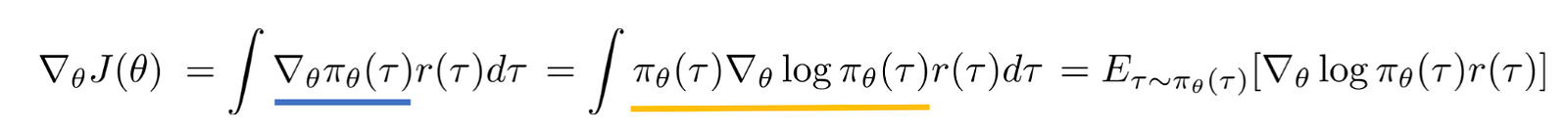# Policy Gradients and Log Derivative Trick

This article will give a high-level picture for those who want to use Reinforcement tricks to solve their ML models but also don’t want to dig too deep into RL field, mainly use Sergey Levine slides for gradient policy method and Shakir Mohamed’s blog for log derivative trick

#### Policy gradient

The goal of the Reinforcement learning is to learn a policy which will give us maximum reward:

The expectation is taken over the trajectories, theta is the parameter of the policy. Policy can be defined as a distribution of the action for a given state. The sum is taken over time steps, at each time step, for a given state, policy tells us which action to take, we get reward, and this action will transit us to the next state, then we sample again an action from the policy on the new state, get the reward and go on…. So the final reward is the sum of the rewards we get at each time step. For simplicity, we do not use the discounted reward here.

To solve such optimization problem, we have to take the derivative of the expectation, which is not as easy as in the case where we optimize empirical loss instead of the true expected loss because we do not know the true data distribution. In the previous case, the parameter we want to optimize does not depend on the sample. However, in the latter, the dependency of the loss and the parameter is only through the samples, once we sample, the loss doesn’t depend on the parameter anymore. Therefore, we can’t just use empirical estimation and get around. Here we have to really deal with the expectation.

If we write the expectation in the integral form and take the derivative with respect to theta:policy gradient

There are two tricks that are used, the first one is the interchange of integral and derivative operations holds under some constraint (for details have to check calculus course)

The second trick is to multiply and divide by the policy. With this two tricks, we are still able to write down gradient of the expectation as an expectation of the gradient so that we can sample the take the gradient and approximate the expectation.

Now the gradient is taken over log probability instead of the probability distribution. When this probability is likelihood then the derivative of log probability is called score function. In fact, if you look carefully at the gradient and compare it with the maximum likelihood gradient, it is the same except we have extra term reward. You can think of it as a weighted gradient with reward. Pushing to move more in the direction of higher reward while less in the direction of less reward.

Now with the above policy gradient equation, we can easily do a gradient update on our policy. So the main steps of the algorithm are:

1. Sample the trajectories from the policy
2. Calculate the gradient
3. Update the policy

If you observe carefully, you will find that the above approach can be used when we have non differentiable functions in your loss. This is one main reason why general ML people want to use policy gradient approaches to solve their problem even though it is not really RL models.

The gradient has high variance and converges very slow.

#### Score function

We have seen the log derivative trick when we derive the policy gradient where we used log derivative trick to bring the derivative of log probability.

When p is likelihood, i.e., when theta is the parameter of the probability distribution of random variable x, this derivative of logarithm of a function p called score function. The most nice property of this function is that its expectation is 0.

The variance is the Fisher information:

#### Score function estimation

It is problematic to compute the gradient of expectation of a function not only because the variable we are taking the derivative with respect to is a parameter of a distribution ( this part re-perametization trick should be able to handle) but also the function f might be non differentiable.

In such case, we can use the score function trick:

Now it should be easy to see that:

Now the non-differentiable function f is not involved in the derivative. The expectation we can calculate it with Monte-Carlo approximation.

This article will be continued on how to solve high variance when we use such estimator.